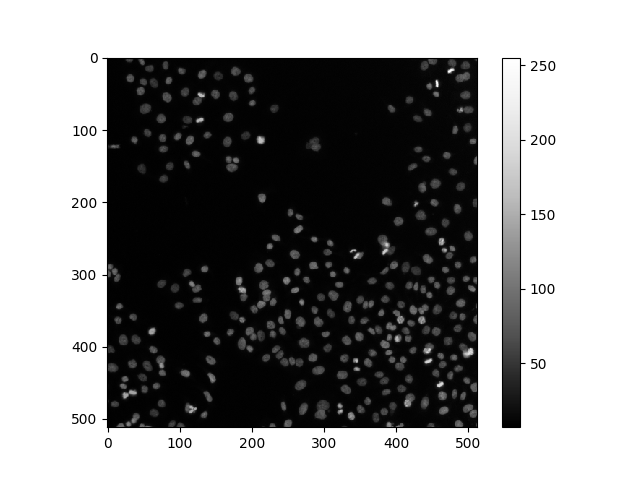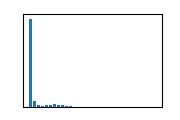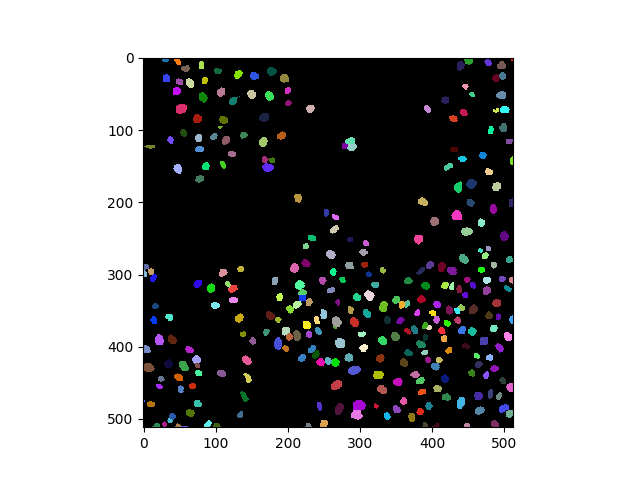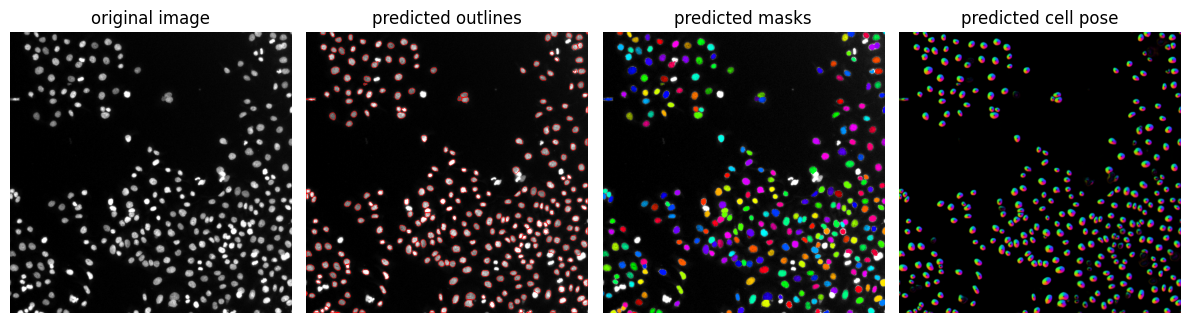# Image Segmentation with CellPose#

CellPose is a deep-learning based segmentation algorithm for cells and nuclei in microscopy images.

import stackview
from cellpose import models, io
import numpy as np
from skimage.data import human_mitosis

image = human_mitosis()
stackview.insight(image)shape (512, 512) dtype uint8 size 256.0 kB min 7 max 255CellPose comes with a number of pretrained models, e.g. for segmenting images showing cells or nuclei. We will just load a model for segmenting nuclei.

model = models.Cellpose(gpu=False, model_type='nuclei')


We let the model “evaluate” the image to produce masks of segmented nuclei.

channels = [0,0] # This means we are processing single-channel greyscale images.

masks, flows, styles, diams = model.eval(image, diameter=None, channels=channels)

stackview.insight(masks.astype(np.uint32))shape (512, 512) dtype uint32 size 1024.0 kB min 0 max 309

## Result visualization#

Cell / nuclei segmentation results can be checked best if the resulting label image is overlaid to the original image or by drawing outlines around segmented regions.

from cellpose import plot
import matplotlib.pyplot as plt

fig = plt.figure(figsize=(12,5))Load ../../data/blobs.tif and apply Cellpose to it.